# 1 Introduction

Simulating data with known properties is an essential step in the development of new statistical methods. Simulating survival data, however, is more challenging than most simulation tasks. To describe this difficulty and our approach to overcoming it, we quote from Harden and Kropko (2018):

Typical methods for generating . . . durations often employ known distributions - such as the exponential, Weibull, or Gompertz — that imply specific shapes for the baseline hazard function. This approach is potentially problematic because it contradicts a key advantage of the Cox model — the ability to leave the distribution of the baseline hazard unspecified. By restricting the simulated data to a known (usually parametric) form, these studies impose an assumption that is not required in applications of the Cox model. In applied research, the baseline hazard in data can exhibit considerable heterogeneity, both across the many fields which employ the Cox model and within a given field . . . . Thus, simulating durations from one specific parametric distribution may not adequately approximate the data that many applied researchers analyze, reducing the simulation’s generalizability.

Here we address these problems by introducing a novel method for simulating durations without specifying a particular distribution for the baseline hazard function. Instead, the method generates — at each iteration of the simulation — a unique baseline hazard by fitting a cubic spline to randomly-drawn points. This produces a wide variety of shapes for the baseline hazard, including those that are unimodal, multimodal, monotonically increasing or decreasing, and other shapes. The method then generates a density function based on each baseline hazard and draws durations accordingly. Because the shape of the baseline hazard can vary considerably, this approach matches the Cox model’s inherent flexibility. By remaining agnostic about the distribution of the baseline hazard, our method better constructs the assumed data generating process (DGP) of the Cox model. Moreover, repeating this process over many iterations in a simulation yields more heterogeneity in the simulated samples of data, which matches the fact that applied researchers analyze a wide variety of data with the Cox model. This increases the generalizability of the simulation results.

In this vignette we demonstrate the uses of the sim.survdata() function for generating survival data. To begin, we load the coxed library:

library(coxed)

# 2 Simulating survival data using the flexible-hazard method

The flexible-hazard method described by Harden and Kropko (2018) first generates a baseline failure CDF: it plots points at (0, 0) and (T+1, 1), and it plots knots additional points with x-coordinates drawn uniformly from integers in [2, T] and y-coordinates drawn from U[0, 1]. It sorts these coordinates in ascending order (because a CDF must be non-decreasing) and if spline=TRUE it fits a spline using Hyman’s (1983) cubic smoothing function to preserve the CDF’s monotonicity. Next it constructs the failure-time PDF by computing the first differences of the CDF at each time point. It generates the survivor function by subtracting the failure CDF from 1. Finally, it computes the baseline hazard by dividing the failure PDF by the survivor function. Full technical details are listed in Harden and Kropko (2018).

## 2.1 Simulating a single dataset

To generate a survival dataset, use the sim.survdata() function, with the num.data.frames argument set to 1. Here we generate a single survival dataset with 1000 observations, in which durations can fall on any integer between 1 and 100:

simdata <- sim.survdata(N=1000, T=100, num.data.frames=1)

## 2.2 Attributes of a simulation

The simulated data has several important attributes:

attributes(simdata)
## $names ##  "data" "xdata" "baseline" "xb" ##  "exp.xb" "betas" "ind.survive" "marg.effect" ##  "marg.effect.data" ## ##$class
##  "simSurvdata"

The full survival dataset, including the durations (y), the censoring variable (failed), and the covariates, is contained in the data frame object stored in the data attribute:

head(simdata$data, 10) ## X1 X2 X3 y failed ## 1 0.170107290 -0.0111568 0.6321666 9 TRUE ## 2 0.304402028 0.1292878 -0.7895115 4 TRUE ## 3 -0.181526802 0.2655182 -0.5265063 34 FALSE ## 4 0.338923058 -0.6961667 -0.5351157 35 TRUE ## 5 0.134959173 -1.1201555 1.1933867 15 FALSE ## 6 0.396404174 -0.2398097 0.1584412 8 TRUE ## 7 -0.404334555 -0.3521984 0.6319572 7 TRUE ## 8 -0.008145304 -0.1120395 1.4398699 38 TRUE ## 9 0.065526183 0.2368340 0.9793140 9 TRUE ## 10 0.101122001 0.2283678 0.5464367 15 FALSE The xdata attribute only contains the covariates: head(simdata$xdata, 10)
##              X1         X2         X3
## 1   0.170107290 -0.0111568  0.6321666
## 2   0.304402028  0.1292878 -0.7895115
## 3  -0.181526802  0.2655182 -0.5265063
## 4   0.338923058 -0.6961667 -0.5351157
## 5   0.134959173 -1.1201555  1.1933867
## 6   0.396404174 -0.2398097  0.1584412
## 7  -0.404334555 -0.3521984  0.6319572
## 8  -0.008145304 -0.1120395  1.4398699
## 9   0.065526183  0.2368340  0.9793140
## 10  0.101122001  0.2283678  0.5464367

The baseline attribute contains the baseline functions – failure PDF, failure CDF, survivor, and hazard – generated by the flexible-hazard method:

head(simdata$baseline, 10) ## time failure.PDF failure.CDF survivor hazard ## 1 1 0.005964947 0.005964947 0.9940351 0.005964947 ## 2 2 0.007864769 0.013829716 0.9861703 0.007911964 ## 3 3 0.009674802 0.023504518 0.9764955 0.009810478 ## 4 4 0.011395046 0.034899564 0.9651004 0.011669328 ## 5 5 0.013025500 0.047925064 0.9520749 0.013496523 ## 6 6 0.014566165 0.062491229 0.9375088 0.015299389 ## 7 7 0.016017041 0.078508270 0.9214917 0.017084684 ## 8 8 0.017378127 0.095886397 0.9041136 0.018858690 ## 9 9 0.018649424 0.114535821 0.8854642 0.020627302 ## 10 10 0.019830932 0.134366754 0.8656332 0.022396087 As we demonstrate below, we can use the survsim.plot() function to plot these baseline functions. The betas attribute contains the coefficients used in the simulation to generate the durations: simdata$betas
##             [,1]
## [1,] -0.05564586
## [2,]  0.02660538
## [3,]  0.02426556

These coefficients are the “true” coefficients in a data generating process, and a survival model such as the Cox proportional hazards model produces estimates of these coefficients. In this case, the Cox model estimates the coefficients as follows:

model <- coxph(Surv(y, failed) ~ X1 + X2 + X3, data=simdata$data) model$coefficients
##           X1           X2           X3
## -0.059577317 -0.007379051  0.092432766

The coefficients and the covariates together form values of the linear predictor, contained in the xb attribute, and exponentiated values of the linear predictor, contained in the exp.xb attribute:

head(cbind(simdata$xb, simdata$exp.xb))
##              [,1]      [,2]
## [1,]  0.005577281 1.0055929
## [2,] -0.032656902 0.9678706
## [3,]  0.004389454 1.0043991
## [4,] -0.050366324 0.9508810
## [5,] -0.008353877 0.9916809
## [6,] -0.024593811 0.9757062

In the course of generating simulations, the flexible-hazard method requires expressing each individual observation’s survivor function. These functions are contained in the rows of the ind.survive attribute, a matrix with N rows and T columns. For example, here’s the survivor function for observation 1:

simdata$ind.survive[1,] ##  1.00000000 0.99400179 0.98609348 0.97636559 0.96490871 0.95181346 ##  0.93717048 0.92107045 0.90360404 0.88486197 0.86493495 0.84391368 ##  0.82188889 0.79900464 0.77561833 0.75214048 0.72898136 0.70655097 ##  0.68525899 0.66551485 0.64772772 0.63230654 0.61966016 0.61019735 ##  0.60432698 0.60099660 0.59844670 0.59602577 0.59308235 0.58896508 ##  0.58302270 0.57460421 0.56305897 0.54773690 0.52798880 0.50316669 ##  0.46502450 0.41271681 0.35668738 0.30735410 0.27510182 0.26084916 ##  0.25559926 0.25484933 0.25467621 0.25346441 0.25017541 0.24377122 ##  0.23321500 0.21747217 0.14930763 0.08937672 0.08701053 0.08485483 ##  0.08289977 0.08113551 0.07955222 0.07814006 0.07688923 0.07578991 ##  0.07483230 0.07400660 0.07330303 0.07271179 0.07222312 0.07182723 ##  0.07151436 0.07127472 0.07109855 0.07097610 0.07089758 0.07085323 ##  0.07083330 0.07082801 0.07082760 0.07082231 0.07080238 0.07075803 ##  0.07067951 0.07055706 0.07038090 0.07014129 0.06982845 0.06943264 ##  0.06894409 0.06835306 0.06764980 0.06682457 0.06586763 0.06476926 ##  0.06351975 0.06210937 0.06052846 0.05876732 0.05681631 0.05466580 ##  0.05230618 0.04663515 0.03528028 0.01934075 0.00000000 The last two attributes, marg.effect and marg.effect.data, are discussed further below. ## 2.3 Simulating multiple datasets In a simulation, researchers generally want to create many iterations of simulated data to draw inferences from the sampling variation that produces each dataset. To generate multiple datasets, set the num.data.frames argument to the desired number of iterations. For example, to create 2 datasets, type: simdata <- sim.survdata(N=1000, T=100, num.data.frames=2) summary(simdata) ## Length Class Mode ## [1,] 9 -none- list ## [2,] 9 -none- list The output is now a list of two objects, where each object contains another version of the attributes described above. For example, to the see the data for the second iteration, type head(simdata[]$data, 10)
##             X1          X2           X3  y failed
## 1  -0.00286286  0.25891551 -0.338288689 97   TRUE
## 2  -0.41579447 -0.72450746  1.245466044  8   TRUE
## 3  -0.97391819 -0.18167443  0.457997304  9   TRUE
## 4   0.92419900  0.45100884  0.007808306 11  FALSE
## 5   0.02773613  0.20232212 -0.002673795 92   TRUE
## 6  -0.20068537 -0.54140501 -0.090831810 64   TRUE
## 7   0.04678707 -0.05263699 -0.585928334 10   TRUE
## 8   0.47294820 -0.44973996  0.679822548 97   TRUE
## 9   0.31750572 -0.07802505  0.044110424 88   TRUE
## 10  0.77242652  0.44660781 -0.094242652  3   TRUE

In the above example we created two datasets to reduce the time needed to compile this vignette, but for research applications this number can be set as large as a researcher wants, even to num.data.frames=1000 for example.

## 2.4 Plotting the baseline functions and histograms

The flexible-hazard method is designed to avoid assuming a particular parametric form for the hazard function, but it still employs a hazard function. To see the baseline functions, including hazard, use the survsim.plot() function. The first argument is the output of the sim.survdata() function. Every simulation iteration produces different durations (and if fixed.hazard=FALSE, different hazard functions), so it is necessary to use the df argument specify which simulation iteration to use when plotting histograms and baseline functions. To see the baseline functions for the first simulation iteration, specify type="baseline" as follows:

survsim.plot(simdata, df=1, type="baseline")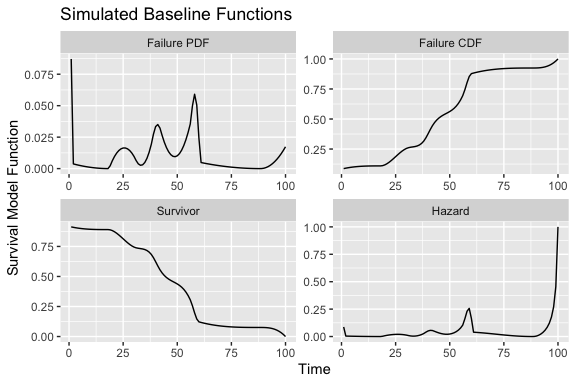To see the histograms of the simulated durations, linear predictors, and exponentiated linear predictors, specify type="hist":

survsim.plot(simdata, df=1, type="hist")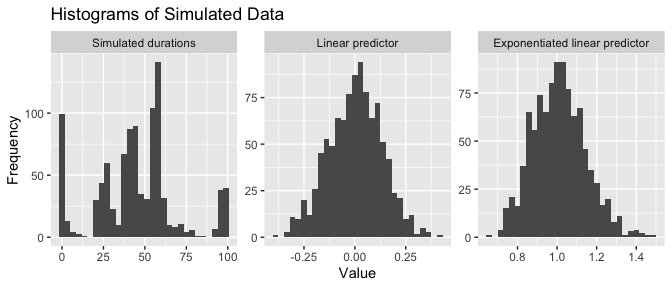And to see both the baseline functions and the histograms, specify type="both":

survsim.plot(simdata, df=1, type="both")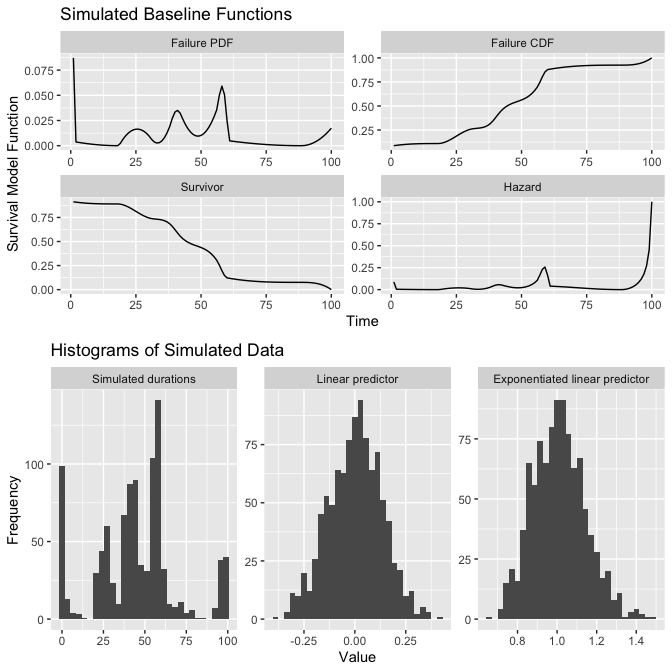## TableGrob (2 x 1) "arrange": 2 grobs
##   z     cells    name           grob
## 1 1 (1-1,1-1) arrange gtable[layout]
## 2 2 (2-2,1-1) arrange gtable[layout]

The survsim.plot() function also has a bins argument, which allows the user to change the number of bins in these histograms (the default is 30).

# 3 Changing simulation parameters

The sim.survdata() function has a number of arguments that allow the user to change parameters of the simulation.

## 3.1 Changing the number of observations, time points, and covariates, and the censoring rate

The N argument changes the number of observations in each simulated dataset, the T argument changes the maximum possible survival time, xvars sets the number of covariates, and the censor argument specifies the proportion of observations that should be designated as right-censored. By default, N=1000, T=100, xvars=3, and censor=.1. To generate data with 700 observations, with durations ranging from 1 to 250, with 5 covariates and 20% of observations right-censored, type:

simdata <- sim.survdata(N=700, T=250, xvars=5, censor=.2, num.data.frames = 1)
summary(simdata$data) ## X1 X2 X3 X4 ## Min. :-1.43988 Min. :-1.29312 Min. :-1.36464 Min. :-1.52276 ## 1st Qu.:-0.33992 1st Qu.:-0.34906 1st Qu.:-0.36720 1st Qu.:-0.32112 ## Median : 0.01321 Median :-0.01082 Median :-0.02187 Median : 0.01739 ## Mean : 0.00410 Mean : 0.01323 Mean :-0.02651 Mean : 0.02603 ## 3rd Qu.: 0.33869 3rd Qu.: 0.35102 3rd Qu.: 0.32255 3rd Qu.: 0.38098 ## Max. : 1.51727 Max. : 1.88520 Max. : 1.52657 Max. : 1.50357 ## X5 y failed ## Min. :-1.35678 Min. : 5.0 Mode :logical ## 1st Qu.:-0.34089 1st Qu.: 65.0 FALSE:129 ## Median :-0.03520 Median :124.0 TRUE :571 ## Mean :-0.01865 Mean :112.4 ## 3rd Qu.: 0.30127 3rd Qu.:164.0 ## Max. : 1.36678 Max. :240.0 ## 3.2 User-supplied coefficients By default the coefficients are drawn from normal distributions, however the user may specify different coefficients with the beta argument if he or she wishes: simdata <- sim.survdata(N=1000, T=100, num.data.frames=1, beta=c(1, -1.5, 2)) ## Warning in FUN(X[[i]], ...): 138 observations have drawn durations ## at the minimum or maximum possible value. Generating coefficients ## and other quantities of interest are unlikely to be returned ## by models due to truncation. Consider making user-supplied coefficients ## smaller, making T bigger, or decreasing the variance of the X variables. simdata$betas
##      [,1]
## [1,]  1.0
## [2,] -1.5
## [3,]  2.0

Another way that a user can specify coefficients is to let these coefficients be dependent on time. An example of that is provided below.

## 3.3 User-supplied data

By default, the covariates are drawn from standard normal distributions, but the sim.survdata() function allows a user to specify different data for the covariates using the X argument. Suppose, for example, that we want to use the data on the length of time needed for governing coalitions in European democracies to conclude negotiations from Martin and Vanberg (2003). The data are stored in the coxed package as martinvanberg:

summary(martinvanberg)
##     formdur           postel          prevdef            cont
##  Min.   :  1.00   Min.   :0.0000   Min.   :0.0000   Min.   :0.0000
##  1st Qu.:  6.00   1st Qu.:0.0000   1st Qu.:1.0000   1st Qu.:0.0000
##  Median : 19.00   Median :1.0000   Median :1.0000   Median :0.0000
##  Mean   : 28.03   Mean   :0.5764   Mean   :0.8522   Mean   :0.3251
##  3rd Qu.: 37.50   3rd Qu.:1.0000   3rd Qu.:1.0000   3rd Qu.:1.0000
##  Max.   :205.00   Max.   :1.0000   Max.   :1.0000   Max.   :1.0000
##      ident           rgovm            pgovno         tpgovno
##  Min.   :1.000   Min.   :0.0000   Min.   :1.000   Min.   : 0.000
##  1st Qu.:1.000   1st Qu.:0.0000   1st Qu.:1.000   1st Qu.: 2.080
##  Median :2.000   Median :0.4200   Median :2.000   Median : 5.890
##  Mean   :2.049   Mean   :0.8009   Mean   :2.261   Mean   : 6.552
##  3rd Qu.:3.000   3rd Qu.:1.4050   3rd Qu.:3.000   3rd Qu.: 8.600
##  Max.   :3.000   Max.   :3.2700   Max.   :6.000   Max.   :25.470
##     minority
##  Min.   :0.0000
##  1st Qu.:0.0000
##  Median :0.0000
##  Mean   :0.3596
##  3rd Qu.:1.0000
##  Max.   :1.0000

We will use the postel, rgovm, and pgovno variables to create a data frame that we pass to the X argument:

mv.data <- dplyr::select(martinvanberg, postel, rgovm, pgovno)
simdata <- sim.survdata(T=100, X=mv.data, num.data.frames = 1)

The data now contain the exogenous covariates and the simulated duration and censoring variables:

head(simdata$data) ## postel rgovm pgovno y failed ## 1 1 3.01 2 92 TRUE ## 2 1 1.95 2 100 TRUE ## 3 1 2.36 2 94 TRUE ## 4 0 2.36 2 92 TRUE ## 5 0 2.36 2 98 TRUE ## 6 1 1.46 2 83 TRUE ## 3.4 Simulated marginal effects The sim.survdata() function also generates a marginal change in duration, conditional on a particular covariate, so that researchers can compare the performance of estimators of this statistic using simulated data. The function calculates simulated durations for each observation conditional on a baseline hazard function and exogenous covariates and coefficients. The argument specifies the variable in the X matrix to vary so as to measure the marginal effect. First the covariate is set to the value specified in for all observations, then to the value specified in for all observations. Given each value, new durations are drawn. The durations when the covariate equals the low value are subtracted from the durations when the covariate equals the high value. The marginal effect is calculated by employing the statistic given by , which is by default. The marginal effect itself is stored in the marg.effect attribute, and the durations and covariates for the high and low profiles are stored as a list in the marg.effect.data attribute. For example, suppose we are interested in comparing the duration when X1 is 1 to the duration when X1 is 0. We specify covariate=1 to indicate that X1 is the variable whose values should be fixed to the ones specified with low=0 and high=1. In this example we fix the coefficients so that X1 has a large effect, to ensure that we see a larger marginal effect. To simulate with these parameters, we type: simdata <- sim.survdata(N=1000, T=100, num.data.frames=1, covariate=1, low=0, high=1, beta = c(2, .1, .1)) ## Warning in FUN(X[[i]], ...): 28 observations have drawn durations ## at the minimum or maximum possible value. Generating coefficients ## and other quantities of interest are unlikely to be returned ## by models due to truncation. Consider making user-supplied coefficients ## smaller, making T bigger, or decreasing the variance of the X variables. The simulated marginal effect is simdata$marg.effect
##  -15

The covariates and durations for each of the low and high conditions are stored in marg.effect.data:

head(simdata$marg.effect.data$low$x) ## X1 X2 X3 ## 1 0 -1.14723722 0.18266086 ## 2 0 0.10268041 0.12169639 ## 3 0 -0.72442473 -0.28856120 ## 4 0 -0.09895171 0.15411065 ## 5 0 -0.23871169 -0.12332868 ## 6 0 0.26712159 0.09054282 head(simdata$marg.effect.data$low$y)
##  28 75  4 17 17 17
head(simdata$marg.effect.data$high$x) ## X1 X2 X3 ## 1 1 -1.14723722 0.18266086 ## 2 1 0.10268041 0.12169639 ## 3 1 -0.72442473 -0.28856120 ## 4 1 -0.09895171 0.15411065 ## 5 1 -0.23871169 -0.12332868 ## 6 1 0.26712159 0.09054282 head(simdata$marg.effect.data$high$y)
##   1  1 17  1 17 17

## 3.5 Fixed or varying hazard

A researcher might want to hold the hazard function fixed from one iteration to the next in order to isolate the effect of different factors. Alternatively, a researcher might want the hazard to vary from one iteration to the next in order to examine a range of possibilities within one analysis and to avoid making any restrictive assumptions about hazard. If fixed.hazard=TRUE, one baseline hazard is generated and the same function is used to generate all of the simulated datasets. If fixed.hazard=FALSE (the default), a new hazard function is generated with each simulation iteration. To illustrate, we create two simulated datasets, twice - once with fixed.hazard=TRUE and once with fixed.hazard=FALSE. If fixed.hazard=TRUE, both data frames yield the same baseline functions:

simdata <- sim.survdata(N=1000, T=100, num.data.frames=2, fixed.hazard=TRUE)
survsim.plot(simdata, df=1, type="baseline")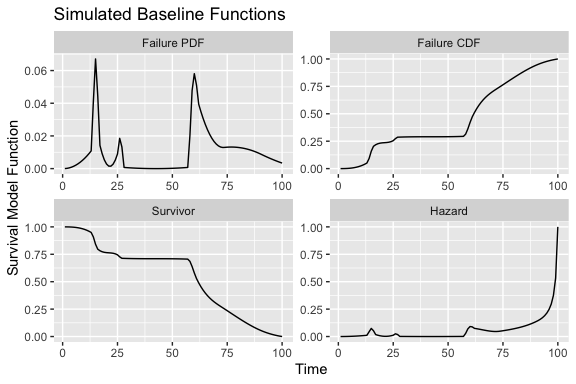survsim.plot(simdata, df=2, type="baseline")If fixed.hazard=FALSE, the two data frames yield different baseline functions:

simdata <- sim.survdata(N=1000, T=100, num.data.frames=2, fixed.hazard=FALSE)
survsim.plot(simdata, df=1, type="baseline")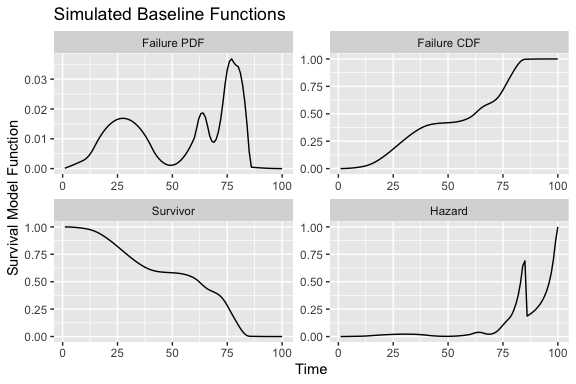survsim.plot(simdata, df=2, type="baseline")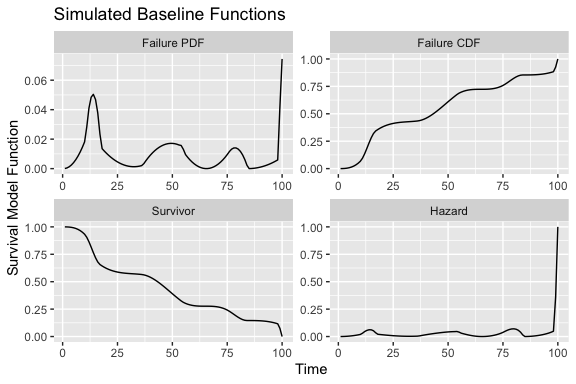## 3.6 Covariate-dependent censoring

An important assumption of many survival models is that the mechanism that causes some observations to be right-censored is independent from the covariates in the model. Some researchers, studying the effects of violations of the assumptions of a model, may want to dispel this assumption in the data generating process to see the effect on the model estimates. In the sim.survdata() function, if censor.cond=FALSE then a proportion of the observations specified by censor is randomly and uniformly selected to be right-censored. If censor.cond=TRUE then censoring depends on the covariates as follows: new coefficients are drawn from normal distributions with mean 0 and standard deviation of 0.1, and these new coefficients are used to create a new linear predictor using the X matrix. The observations with the largest (100 x censor) percent of the linear predictors are designated as right-censored. In other words, if censor.cond=FALSE, then a logit model in which the censoring indicator is regressed on the covariates should yield null results:

simdata <- sim.survdata(N=1000, T=100, num.data.frames=1, censor.cond=FALSE)
logit <- glm(failed ~ X1 + X2 + X3, data=simdata$data, family=binomial(link="logit")) summary(logit) ## ## Call: ## glm(formula = failed ~ X1 + X2 + X3, family = binomial(link = "logit"), ## data = simdata$data)
##
## Deviance Residuals:
##     Min       1Q   Median       3Q      Max
## -2.3573   0.3876   0.4062   0.4244   0.4888
##
## Coefficients:
##             Estimate Std. Error z value Pr(>|z|)
## (Intercept)  2.43772    0.11688  20.857   <2e-16 ***
## X1          -0.24045    0.23184  -1.037    0.300
## X2          -0.10466    0.23516  -0.445    0.656
## X3          -0.01257    0.22633  -0.056    0.956
## ---
## Signif. codes:  0 '***' 0.001 '**' 0.01 '*' 0.05 '.' 0.1 ' ' 1
##
## (Dispersion parameter for binomial family taken to be 1)
##
##     Null deviance: 562.41  on 999  degrees of freedom
## Residual deviance: 561.13  on 996  degrees of freedom
## AIC: 569.13
##
## Number of Fisher Scoring iterations: 5

If, however, censor.cond=TRUE, then a logit model in which the censoring indicator is regressed on the covariates should yield significant results:

simdata <- sim.survdata(N=1000, T=100, num.data.frames=1, censor.cond=TRUE)
logit <- glm(failed ~ X1 + X2 + X3, data=simdata$data, family=binomial(link="logit")) summary(logit) ## ## Call: ## glm(formula = failed ~ X1 + X2 + X3, family = binomial(link = "logit"), ## data = simdata$data)
##
## Deviance Residuals:
##      Min        1Q    Median        3Q       Max
## -3.00252   0.08653   0.19870   0.38207   1.64839
##
## Coefficients:
##             Estimate Std. Error z value Pr(>|z|)
## (Intercept)   3.4869     0.2239  15.574  < 2e-16 ***
## X1            3.1663     0.3422   9.252  < 2e-16 ***
## X2            0.9282     0.2637   3.519 0.000433 ***
## X3           -2.4473     0.3033  -8.068 7.14e-16 ***
## ---
## Signif. codes:  0 '***' 0.001 '**' 0.01 '*' 0.05 '.' 0.1 ' ' 1
##
## (Dispersion parameter for binomial family taken to be 1)
##
##     Null deviance: 650.17  on 999  degrees of freedom
## Residual deviance: 437.89  on 996  degrees of freedom
## AIC: 445.89
##
## Number of Fisher Scoring iterations: 7

## 3.7 User-specified hazard functions

For some applications, a researcher may want to specify his or her own hazard function rather than relying on the flexible-hazard method of generating hazard functions and drawing durations. The sim.survdata() allows a user to specify a customized hazard function with the hazard.fun argument, which takes a function with one argument that represents time. For example, suppose we want a lognormal hazard function with a mean of 50 and a standard deviation of 10. We can specify that function as follows:

my.hazard <- function(t){
dnorm((log(t) - log(50))/log(10)) /
(log(10)*t*(1 - pnorm((log(t) - log(50))/log(10))))
}

We can then pass that function to sim.survdata(). Note that the lognormal hazard function does not equal 0 when T=100. That means that many observations will be naturally right-censored above and beyond the amount of right-censoring we’ve specified with the censor argument (a proportion of .1 by default), simply because we haven’t allowed the simulated durations to extend to later time points. To reduce the number of additional right-censored observations, we increase T to 1000 to allow for the longer durations that the log-normal hazard function implies. Note, the sim.survdata() produces a warning with the number of additional right-censored observations:

simdata <- sim.survdata(N=1000, T=1000, num.data.frames=1, hazard.fun = my.hazard)
## Warning in FUN(X[[i]], ...): 94 additional observations right-censored because the user-supplied hazard function
##                                   is nonzero at the latest timepoint. To avoid these extra censored observations, increase T

To see the hazard, we can use the simsurv.plot() function with type="baseline":

survsim.plot(simdata, df=1, type="baseline")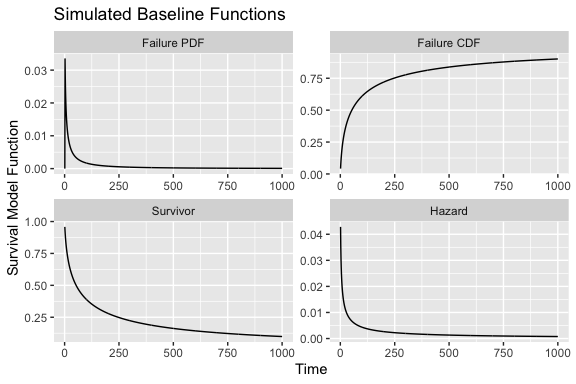## 3.8 Time-varying covariates

Time-varying covariates require a different data structure. The Surv() function in the survival package sets up the dependent variable for a Cox model. Generally it has two arguments: duration time and a censoring variable. But for time-varying covariates it replaces the duration argument with two time arguments, representing the start and end of discrete intervals, which allows a covariate to take on different values across different intervals for the same observation.

The sim.survdata() function generates data with a time-varying covariate if type="tvc". Durations are drawn again using proportional hazards, and are passed to the permalgorithm() function in the PermAlgo package to generate the time-varying data structure (Sylvestre and Abrahamowicz 2008). If type="tvc", the sim.survdata() function cannot accept user-supplied data for the covariates, as a time-varying covariate is expressed over time frames which themselves convey part of the variation of the durations, and we are generating these durations. If user-supplied X data is provided, the function passes a warning and generates random data instead as if . To generate survival data with 1000 observations, a maximum duration of 100, and time-varying covariates, type:

simdata <- sim.survdata(N=1000, T=100, type="tvc", num.data.frames=1)
head(simdata$data, 20) ## id failed start end X1 X2 X3 ## 1 1 0 0 1 -1.90606234 -1.531762 0.8464572 ## 2 1 0 1 2 -0.21630326 -1.531762 0.8464572 ## 3 1 0 2 3 -0.76871752 -1.531762 0.8464572 ## 4 1 0 3 4 -0.07674372 -1.531762 0.8464572 ## 5 1 0 4 5 -0.22631307 -1.531762 0.8464572 ## 6 1 0 5 6 1.54577695 -1.531762 0.8464572 ## 7 1 0 6 7 -1.02933124 -1.531762 0.8464572 ## 8 1 0 7 8 0.08048104 -1.531762 0.8464572 ## 9 1 0 8 9 0.73110892 -1.531762 0.8464572 ## 10 1 0 9 10 -1.53499618 -1.531762 0.8464572 ## 11 1 0 10 11 1.54585825 -1.531762 0.8464572 ## 12 1 0 11 12 0.16796923 -1.531762 0.8464572 ## 13 1 0 12 13 -1.75037803 -1.531762 0.8464572 ## 14 1 0 13 14 0.46092379 -1.531762 0.8464572 ## 15 1 0 14 15 -0.74732030 -1.531762 0.8464572 ## 16 1 0 15 16 0.46000805 -1.531762 0.8464572 ## 17 1 0 16 17 -1.86022722 -1.531762 0.8464572 ## 18 1 0 17 18 1.11926430 -1.531762 0.8464572 ## 19 1 0 18 19 1.13039373 -1.531762 0.8464572 ## 20 1 0 19 20 0.89752863 -1.531762 0.8464572 ## 3.9 Time-varying coefficients The Cox model assumes proportional hazards, and one way to dispel this assumption is to allow the coefficients to vary over time. The sim.survdata() function generates data using time-varying coefficients if the type="tvbeta" argument is specified. If this option is specified, the first coefficient, whether coefficients are user-supplied or randomly generated, is interacted with the natural log of the time counter from 1 to T (the maximum possible duration). Durations are generated via proportional hazards, and coefficients are saved as a matrix to illustrate their dependence on time. To generate data with time-varying coefficients, type: simdata <- sim.survdata(N=1000, T=100, type="tvbeta", num.data.frames = 1) The coefficients are saved as a matrix with a column to represent time: head(simdata$betas, 10)
##    time      beta1      beta2       beta3
## 1     1 0.00000000 -0.1010487 -0.04181902
## 2     2 0.09355976 -0.1010487 -0.04181902
## 3     3 0.14828871 -0.1010487 -0.04181902
## 4     4 0.18711952 -0.1010487 -0.04181902
## 5     5 0.21723903 -0.1010487 -0.04181902
## 6     6 0.24184847 -0.1010487 -0.04181902
## 7     7 0.26265545 -0.1010487 -0.04181902
## 8     8 0.28067928 -0.1010487 -0.04181902
## 9     9 0.29657742 -0.1010487 -0.04181902
## 10   10 0.31079879 -0.1010487 -0.04181902

An alternative approach to specifying time-varying coefficients is for the user to supply the matrix of time-varying coefficients. With this approach the coefficient matrix must have the same number of rows as the maximum duration specified with T, which in this case is 100. Suppose that we specify three coefficients for three covariates, over the time frame from 1 to 100. We want the first coefficient to be given by $\beta_{1_t} = \frac{(t - 25)^2}{2500},$ and the second and third coefficients to be given by .5 and -.25 respectively:

beta.mat <- data.frame(beta1 = (1:100 - 25)^2/2500,
beta2 = .5,
beta3 = -.25)
head(beta.mat)
##    beta1 beta2 beta3
## 1 0.2304   0.5 -0.25
## 2 0.2116   0.5 -0.25
## 3 0.1936   0.5 -0.25
## 4 0.1764   0.5 -0.25
## 5 0.1600   0.5 -0.25
## 6 0.1444   0.5 -0.25

We pass this matrix to the sim.survdata() function through the beta argument:

simdata <- sim.survdata(N=1000, T=100, type="tvbeta", beta=beta.mat, num.data.frames = 1)
## Warning in FUN(X[[i]], ...): 244 observations have drawn durations
##                                     at the minimum or maximum possible value. Generating coefficients
##                                     and other quantities of interest are unlikely to be returned
##                                     by models due to truncation. Consider making user-supplied coefficients
##                                     smaller, making T bigger, or decreasing the variance of the X variables.

The data from this simulation are as follows:

head(simdata$data, 10)  ## X1 X2 X3 y failed ## 1 -0.36817808 -0.23254342 -0.57804004 58 TRUE ## 2 -0.03694465 -0.28627668 0.99227449 72 TRUE ## 3 -0.98317666 0.35188983 -0.13162880 100 TRUE ## 4 0.37622072 0.42199100 -0.15888307 42 TRUE ## 5 0.59217269 -0.57079143 0.08439451 41 TRUE ## 6 -0.38121357 0.02067146 -0.03343044 100 TRUE ## 7 0.45292897 -0.04320535 -0.14226708 79 TRUE ## 8 -0.79528695 -0.59437353 -0.23636561 100 TRUE ## 9 -0.71618017 -0.08681117 0.58447233 58 TRUE ## 10 -0.31398211 -0.71822103 0.34565131 100 TRUE And the coefficients are as we specified earlier: head(simdata$betas, 10) 
##    time  beta1 beta2 beta3
## 1     1 0.2304   0.5 -0.25
## 2     2 0.2116   0.5 -0.25
## 3     3 0.1936   0.5 -0.25
## 4     4 0.1764   0.5 -0.25
## 5     5 0.1600   0.5 -0.25
## 6     6 0.1444   0.5 -0.25
## 7     7 0.1296   0.5 -0.25
## 8     8 0.1156   0.5 -0.25
## 9     9 0.1024   0.5 -0.25
## 10   10 0.0900   0.5 -0.25